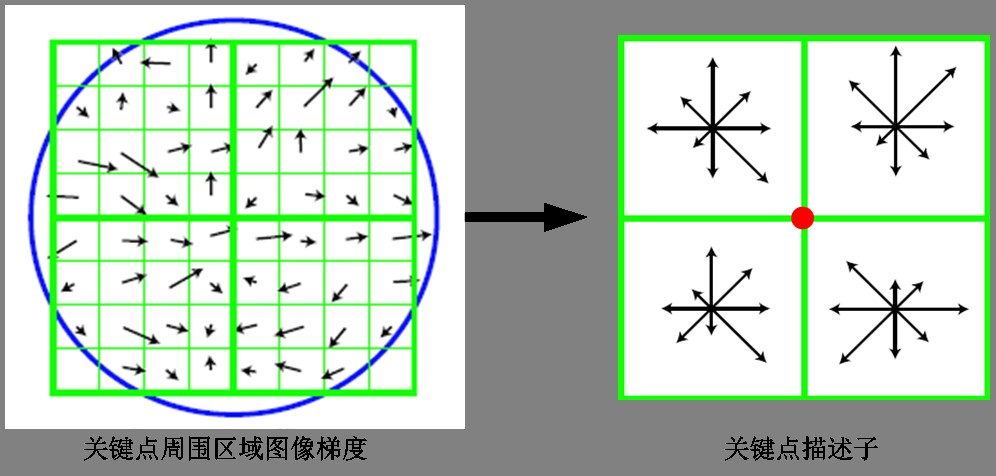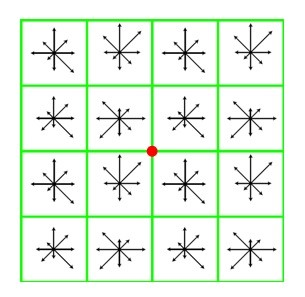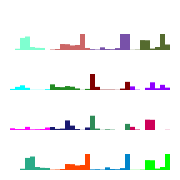# SIFT特征详解

## 1 概览

SIFT特征即Scale-Invariant Feature Transform，是一种用于检测和描述数字图像中的局部特征的算法。它定位关键点并以量化信息呈现（所以称之为描述器），可以用来做目标检测。此特征可以被认为可以对抗不同变换（即同一个特征在不同变换下可能看起来不同）而保持不变。

SIFT特征的提取步骤

1. 生成高斯差分金字塔（DOG金字塔），尺度空间构建
2. 空间极值点检测（关键点的初步查探）
3. 稳定关键点的精确定位
4. 稳定关键点方向信息分配
5. 关键点描述
6. 特征点匹配

## 2 生成差分高斯金字塔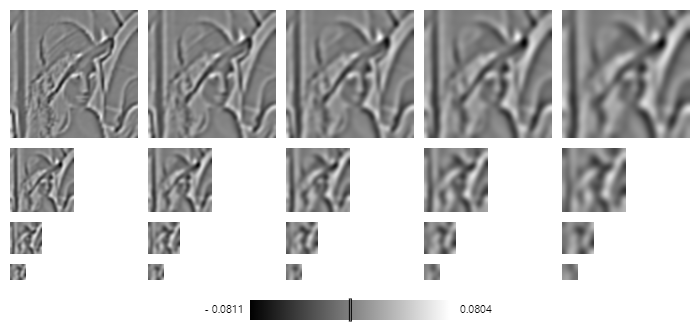## 3 空间极值点检测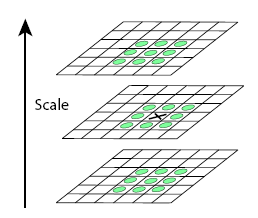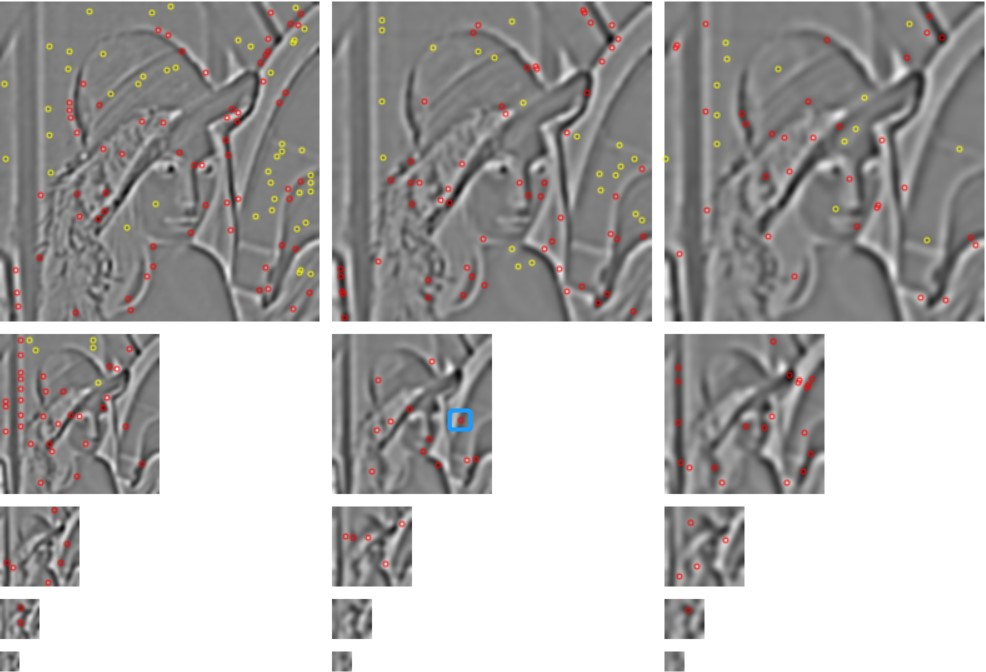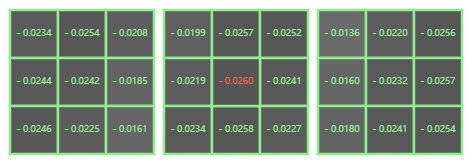## 4 稳定关键点的精确定位

1. 空间尺度函数泰勒展开式如下： $D(x)=D+\frac{\partial D^T}{\partial x}x+\frac{1}{2}x^T\frac{\partial ^2D}{\partial x^2}x \\ 对上式求导，令其为0，得到精确地位置，有\\ \hat x=-\frac{\partial ^2D^{-1}}{\partial x^2}\frac{\partial D}{\partial x}$

2. 在已经检测到的特征点中，要去掉低对比度的特征点和不稳定地边缘响应点。去除低对比度的点：把$\hat x$的值代回，即在Dog 空间的极值点$D(x)$处取值，只取前两项可得： $D(\hat x)=D+\frac{1}{2}\frac{\partial D^T}{\partial x}\hat x$ 若 $|D(\hat x)\ge 0.003$，该特征点就保留下来，否则丢弃

3.边缘响应的去除。一个定义不好的高斯差分算子的极值在横跨边缘的地方有较大的曲率，而在垂直边缘的方向有较小的曲率。主曲率通过一个$2\times 2$的Hessian矩阵H去求出： $% $ 使用python计算hessian矩阵的代码可以参考

import numpy as np

def hessian(x):
"""
Calculate the hessian matrix with finite differences
Parameters:
- x : ndarray
Returns:
an array of shape (x.dim, x.ndim) + x.shape
where the array[i, j, ...] corresponds to the second derivative x_ij
"""
hessian = np.empty((x.ndim, x.ndim) + x.shape, dtype=x.dtype)
# 遍历每个维度，在一阶导数的所有项再次使用梯度
hessian[k, l, :, :] = grad_kl
return hessian

x = np.random.randn(100, 100, 100)
hessian(x)


## 5 给特征点赋值一个128维方向参数

• 其梯度幅值表述为：
• 梯度方向为： $\theta (x,y) = tan ^{-1}[\frac{L(x,y+1)-L(x,y-1)}{L(x+1,y)-L(x-1,y)}]$

1. 计算关键点为中心邻域内所有点的梯度方向。0-360度
2. 每个方向10度，共36个方向。
3. 统计累计落在每个方向点的关键点个数，依次生成梯度直方图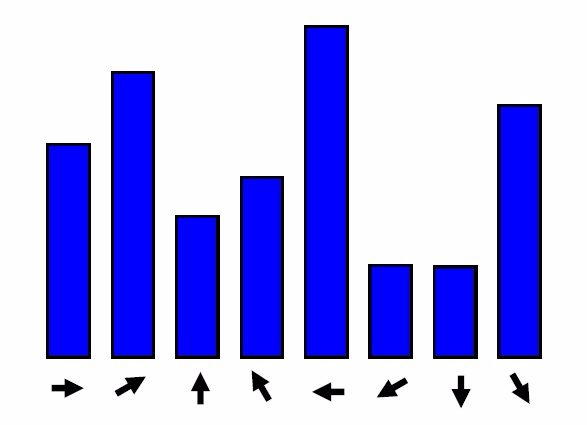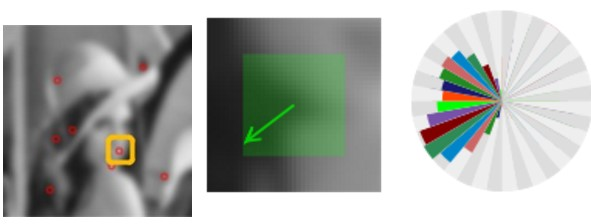## 6 计算SIFT特征描述子